Courses

#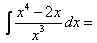a)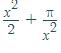where C is the constant of integrationb)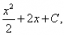where C is the constant of integrationc)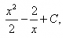where C is the constant of integrationd)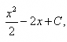where C is the constant of integrationCorrect answer is option 'A'. Can you explain this answer?

## JEE Question

By Manish Lohiya · 2 weeks ago ·JEE
Amrendra_ Official answered 3 weeks ago
C part with no negative sign

Anand Kumar answered 6 days ago
{[(x^4 - 2x)/x^3] dx = {x dx - {[2/(x^2)]dx

= [(x^2/2) + (2/x)] + C